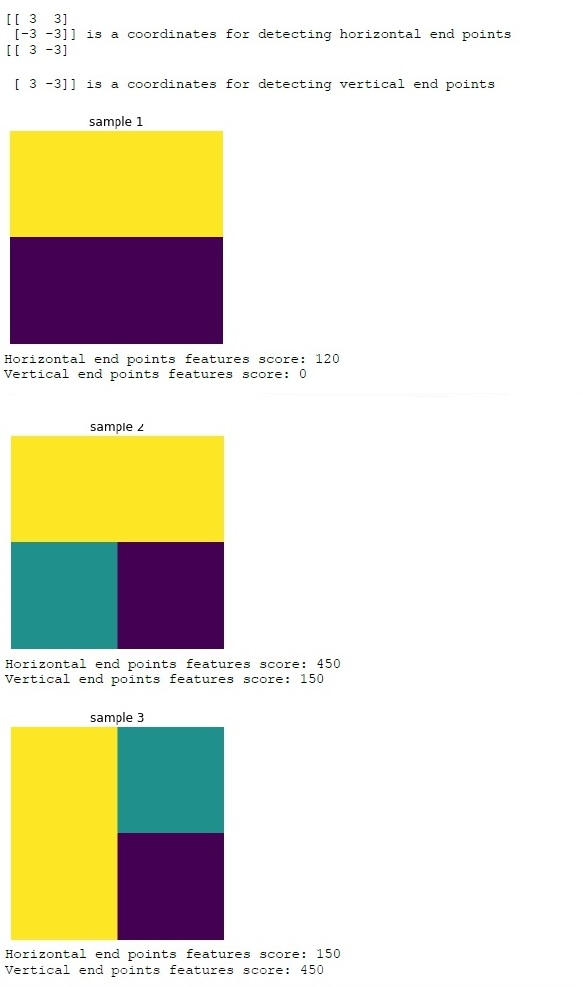# Introduction-to-convolutions-using-python

Ide preferred − Jupyter notebook

Prerequisites − Numpy installed, Matplotlib installed

## Installation

>>> pip install numpy
>>>pip install matplotlib

## Convolution

Convolution is a type of operation that can be performed on an image to extract the features from it by applying a smaller container called a kernel/coordinate container like a sliding window over the image. Depending on the values in the convolutional coordinate container, we can pick up specific patterns/features from the image.Here, we will learn about the detection of horizontal and vertical endpoints in an image using appropriate coordinate containers.

Now let’s see the practical implementation.

## Example

import numpy as np
from matplotlib import pyplot
# initializing the images
img1 = np.array([np.array([100, 100]), np.array([80, 80])])
img2 = np.array([np.array([100, 100]), np.array([50, 0])])
img3 = np.array([np.array([100, 50]), np.array([100, 0])])
coordinates_horizontal = np.array([np.array([3, 3]), np.array([-3, -3])])
print(coordinates_horizontal, 'is a coordinates for detecting horizontal end points')
coordinates_vertical = np.array([np.array([3, -3]), np.array([3, - 3])])
print(coordinates_vertical, 'is a coordinates for detecting vertical end points')
#his will be an elemental multiplication followed by addition
def apply_coordinates(img, coordinates):
return np.sum(np.multiply(img, coordinates))
# Visualizing img1
pyplot.imshow(img1)
pyplot.axis('off')
pyplot.title('sample 1')
pyplot.show()
# Checking for horizontal and vertical features in image1
print('Horizontal end points features score:',
apply_coordinates(img1, coordinates_horizontal))
print('Vertical end points features score:',
apply_coordinates(img1,coordinates_vertical))
# Visualizing img2
pyplot.imshow(img2)
pyplot.axis('off')
pyplot.title('sample 2')
pyplot.show()
# Checking for horizontal and vertical features in image2
print('Horizontal end points features score:',
apply_coordinates(img2, coordinates_horizontal))
print('Vertical end points features score:',
apply_coordinates(img2, coordinates_vertical))
# Visualizing img3
pyplot.imshow(img3)
pyplot.axis('off')
pyplot.title('sample 3')
pyplot.show()
# Checking for horizontal and vertical features in image1
print('Horizontal end points features score:',
apply_coordinates(img3,coordinates_horizontal))
print('Vertical end points features score:',
apply_coordinates(img3,coordinates_vertical))

## Output## Conclusion